A thermistor is an electronic component that displays a substantial change in resistance when there is a change in its body temperature. The term ‘thermistor’ is an amalgamation of the words ‘thermal resistor’. Thermistors are available in two types - NTC and PTC.

What are NTC Thermistors?

NTC Thermistors are a type of thermistors that possess a negative temperature coefficient. This means that where resistance drops, temperature increases (Figure 1).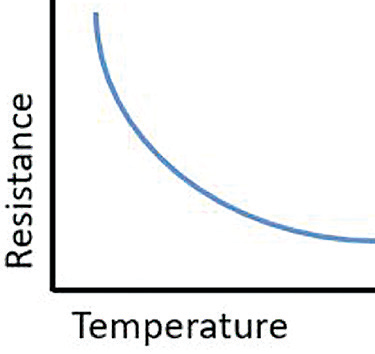Figure 1.

## What are Some NTC Thermistor Applications?

NTC thermistors are useful in a range of settings and applications, including:

Temperature Measurements: A thermistor’s high sensitivity makes it an ideal option for cost-effective temperature measurement applications.

Temperature Control: It is possible to employ NTC thermistors for temperature control (on/off), with this only requiring a small amount of circuitry.

Temperature Compensation: Circuit precision generally involves some sort of temperature compensation being required. Examples of circuits that may need temperature compensation include LCD displays, oscillators, a battery under charge or some models of amplifier.

Inrush Current Limiter: When an NTC disc thermistor is subjected to a variation in power, it will experience a time lag before a lower resistance is reached. The resulting time lag can be used to limit the inrush surge current with larger parts resulting in greater lag.

Fluid Level Applications: It is possible to employ NTC thermistors to sense the presence or absence of a liquid. This can be achieved by utilizing the difference in dissipation constants between a gas and a liquid.

## What are PTC Thermistors?

PTC Thermistors are thermistors which possess a positive temperature coefficient. This means that resistance increases as temperature increases (Figure 2).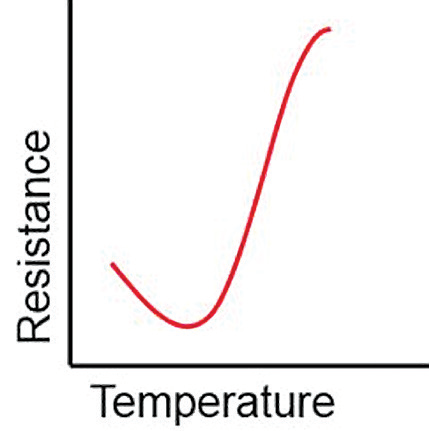Figure 2.

## What are Some PTC Thermistor Applications?

Overcurrent Protection: If a fault condition arises, the PTC thermistor will heat up, prompting a change in resistance from low to very high.

Electronic Ballast Design: PTC thermistors are employed in electronic ballast systems, functioning as a time delay element when a circuit is energized.

Motor Starting: Where a PTC thermistor is installed in series with the starting windings of a single phase electronic motor, the PTC is able to function as a time delay.

Battery Management: When a rechargeable battery is fully charged, its temperature increases. This causes an increase in PTC resistance, quickly reducing the charge to a low level.

Self-Regulating Heater: A PTC thermistor is able to provide a combination of thermostat and heater in a single device. PTC resistance rises as ambient temperature increases; resulting in a power reduction to the circuit.

## What are the Individual Parameters of a Thermistor?

Thermistors operate via a range of parameters. These include Zero-load Resistance Value, Beta (β) Constant, Ratio, Alpha (α), Thermal Time Constant (τ) and Dissipation Constant (δ). The function of each of these parameters is outlined below.

## What is the Zero-Load Resistance Value?

The Zero-load Resistance Value represents a thermistor’s resistance value as measured at a specified temperature. This is also known as the no-load resistance value. This value is typically measured at a standard temperature of 25 °C (R25), with the measurement performed at a power level which results in negligible influence from spontaneous heat generation.

## What is Beta (β) Constant?

Figure 3 displays the Beta Constant. This is the slope of the NTC thermistor’s resistance to temperature characteristic (in kelvins), shown over a particular temperature range. This is illustrated via the formula: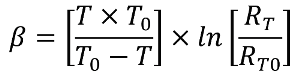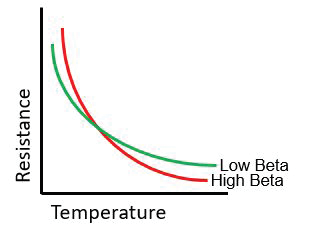Figure 3.

## What is the Ratio?

Ratio in this context is the ratio of a themistor’s resistance at two separate temperature points.

## What is the Alpha (α)?

The Alpha represents the zero-power temperature coefficient of resistance. This is the ratio at a specific temperature, of the rate of change of zero-power resistance, with temperature to the zero-power resistance of the thermistor. In basic terms, the Alpha is the % variation in resistance per °C change in temperature at a specific temperature.

## What is the Thermal Time Constant (τ)?

Figure 4 displays the Thermal Time Constant. This is the time needed for a thermistor to change 63.2 % of the total difference between the thermistor’s start and end body temperature when exposed to a step function temperature change under zero-power conditions.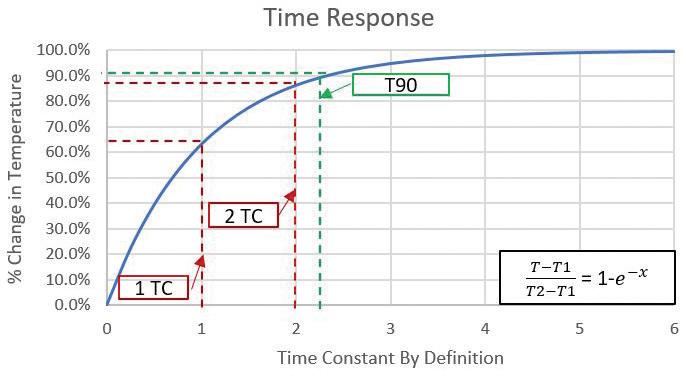Figure 4.

## What is the Dissipation Constant (δ)?

The Dissipation Constant is the ratio (measured in milliwatts per °C) at a particular ambient temperature, of a change in power dissipation in a thermistor to the resultant body temperature change. Fundamentally, the Dissipation Constant represents the power needed to change the thermistor by 1 °C via self-heating.This information has been sourced, reviewed and adapted from materials provided by Amphenol Advanced Sensors.

## Citations

• APA

Amphenol Advanced Sensors. (2020, June 12). Frequently Asked Questions About Thermistors. AZoSensors. Retrieved on July 05, 2020 from https://www.azosensors.com/article.aspx?ArticleID=1964.

• MLA

• Chicago